# Algebra Review - Strengthening Algebraic Skills for Higher Math, Science, Finance

Sale ends in
Teacher: Christina
Customers Who Have Viewed This Course: 1812
\$200.00
\$95.01

#### 0 Introduction and Overview for Algebra Review - Basic Algebraic Skills 02:02

Provides an introduction and overview for the Algebra Review - Basic Algebraic Skills course.  Introduces the content, why it is important, and where it can be used.

#### 1 Combining Like Terms 05:10This is a quick review on how to combine like terms and reminds how to add and subract integers.  Includes adding constants that can be integers, rational numbers, irrational numbers and more.  And combines terms with like variables.

#### 2 Combining Like Terms Homework Answers 01:20

Presents the answers to the Combining Like Terms Lesson Homework by demonstrating how to solve homework problems.

#### 3 Order of Operations 07:41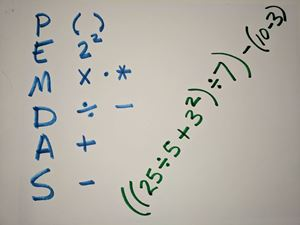Reviews the order of operations.  Solving a numberical or algebraic expression can have different results if the order of operations is not followed.  The order must follow PEMDAS which stands for parenthesis, exponenents, multiplication, division, addition, then finally subtraction.

#### 4 Order of Operations Homework Answers 12:13

Presents the answers to the Order of Operations Lesson Homework by demonstrating how to solve homework problems.

#### 5 Evaluating Algebraic Expressions 06:42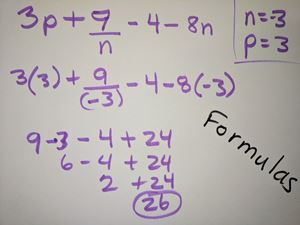Reviews how to evaluate algebraic expressions by plugging in given values for the variables.  Care must be taken to keep track of negative signs and to follow the order of operations.  This skill of plugging in numbers and evaluating to get an answer is critical for utilizing formulas in finance classes, science classes such as physics and chemistry, and more.

#### 6 Evaluating Algebraic Expressions Homework Answers 08:56

Presents the answers to the Evaluating Algebraic Expressions Lesson Homework by demonstrating how to solve homework problems.

#### 7 Simplifying Exponential Expressions 08:42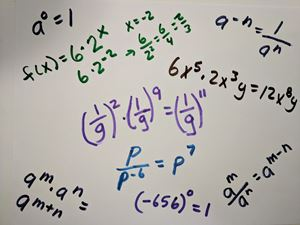Simplify exponential expressions including zero exponents, negative exponents, multiplying and dividing when two variables have same base.

#### 8 Simplifying Exponential Expressions Homework Answers 06:19

Presents the answers to the Simplifying Exponential Expressions Lesson Homework by demonstrating how to solve homework problems.

#### 9 Distributive Property 06:00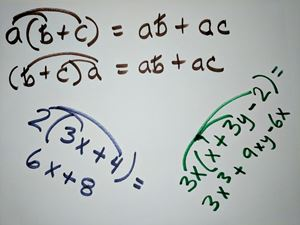Reviews the distributive property used to mulitiply across algebraic expressions.

#### 10 Distributive Property Homework Answers 04:46

Presents the answers to the Distributive Property Lesson Homework by demonstrating how to solve homework problems.

#### 11 Multiplying Binomial Expressions and FOIL 10:23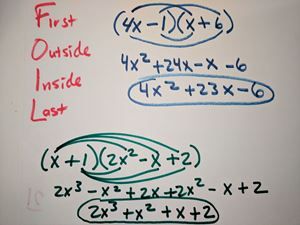Review distributive property by extending to multiplying two binomial expressions using the FOIL method. Foil stands for first, outside, inside, and last. Show how to also use the distributive property to multiply a binomial and a trinomial.

#### 12 Multiplying Binomial Expressions and FOIL Homework Answers 05:41

Presents the answers to the Multiplying Binomial Expressions and FOIL Lesson Homework by demonstrating how to solve homework problems.

#### 13 Factoring 14:08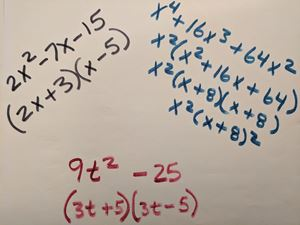Reviews factoring of algebraic expressions.  Includes factoring special cases.

This lesson has 2 homework sheets and will take longer to complete than those with a single homework sheet.

#### 14 Factoring Homework Part 1 Answers 04:07

Presents the answers to the Factoring Lesson Homework Part 1 by demonstrating how to solve homework problems.

#### 15 Factoring Homework Part 2 Answers 06:16

Presents the answers to the Factoring Lesson Homework Part 2 by demonstrating how to solve homework problems.

#### 17 Writing Variable Expressions 07:23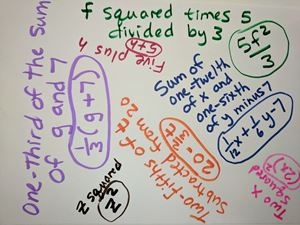Reviews how to translate word phrases into algebraic variable expressions.  Includes arranging addition, subtraction, multiplication, division, fractional parts, and exponents.

#### 18 Writing Variable Expressions Homework Answers 01:50

Presents the answers to the Writing Variable Expressions Lesson Homework by demonstrating how to solve homework problems.

#### 19 Solving Algebraic Equations 09:30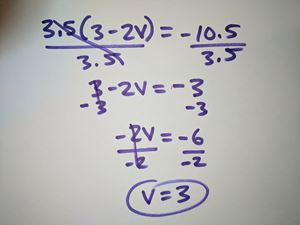Reviews how to solve algebraic expressions to find the value of the variable.  Includes integer and decimal coefficients and a trick to solving proportions quickly.

This lesson has 3 homework sheets and will take longer to complete than those with a single homework sheet.

#### 20 Solving Algebraic Equations Homework Part 1 Answers 06:34

Presents the answers to the Solving Algebraic Equations Lesson Homework Part 1 by demonstrating how to solve homework problems.

#### 21 Solving Algebraic Equations Homework Part 2 Answers 21:12

Presents the answers to the Solving Algebraic Equations Lesson Homework Part 2 by demonstrating how to solve homework problems.

#### 22 Solving Algebraic Equations Homework Part 3 Answers 09:30

Presents the answers to the Solving Algebraic Equations Lesson Homework Part 3 by demonstrating how to solve homework problems.

#### 23 Solving Inequalities 06:53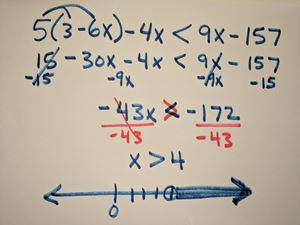Review solving of algebraic equations involving inequalities and graph on the number line.

This lesson has 2 homework sheets and will take longer to complete than those with a single homework sheet.

#### 24 Solving Inequalities Homework Part 1 Answers 14:01

Presents the answers to the Solving Inequalities Homework Part 1 by demonstrating how to solve homework problems.

#### 25 Solving Inequalities Homework Part 2 Answers 11:05

Presents the answers to the Solving Inequalities Lesson Homework Part 2 by demonstrating how to solve homework problems.

#### 26 Absolute Value 05:28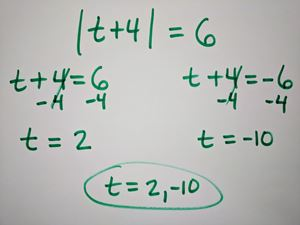Reviews absolute value and solving of algebraic equations involving absolute value.

#### 27 Absolute Value Homework Answers 10:32

Presents the answers to the Absolute Value Lesson Homework by demonstrating how to solve homework problems.

This module provides a review of basic algebraic skills needed to be successful in advanced algebra or any other course that requires algebra as a pre-requisite.

Reviews

Includes a review of the following skills:  combining like terms, order of operations, evaluating algebraic expressions, simplifying exponential expressions, distributive property, multiplying binonomial expressions and FOIL, factoring, writing variable expressions, solving algebraic expressions, solving inequalities, and absolute value.

Course Goals

Intended to review and strengthen basic Algebra skills needed for courses taken after successful completion of Algebra.

Target Audience

This course is intended for student that have completed an Algebra course, as a review of Algebra, and is recommended for any student preparing to take any course that requires Algebra as a pre-requisite, such as Geometry, or Advanced Algebra students such as Math for 10th Grade.  Also valuable for Finance, Statistics, Chemistry, Physics.  Can also be taken at anytime during a course when the need for review of basic Algebra is observed.

Pre-requisites

Successful completion of Algebra course.

Course Lessons

- Combining like terms

- Order of operations

- Evaluating algebraic expressions

- Simplifying exponential expressions

- Distributive property

- Multiplying binonomial expressions and FOIL

- Factoring

- Writing variable expressions

- Solving algebraic expressions

- Solving inequalities

- Absolute value.

Resources

15 Homework Worksheets

15 Homework Worksheets Keys

• Teacher: Christina
• Areas of expertise: Secondary Mathematics - Grades 7-12, including Basic Math, Algebra, Geometry, Trigonomety, Pre-Calculus, Calculus. Physical Science such as Physics and Chemistry
• Education: Michigan Professional Teacher Certification-Math,Physics,Chemistry; Bachelor of Science-Mechanical Engineering; Master of Science-Packaging of Electronic and Optical Equipment
• Interests: Math application in the real world.
• Skills: Presentation
• Associations:
• Issues I care about: Using creative and varied ways to make challenging subject understable to everyone.

Everyone should have the opportunity to learn challenging subjects. It is my job to make it understandable.

#### Digital Quizzes and Tests Answer Keys Document

Answers to the quizzes that are part of the homework answers lessons.

#### Combining Like Terms Homework Key

Provides the answers to the homework worksheet for Combining Like Terms.

#### Order of Operations Homework Key

Provides the answers to the homework worksheet for Order of Operations.

#### Evaluating Algebraic Expressions Homework Key

Provides the answers to the homework worksheet for Evaluating Algebraic Expressions.

#### Simplifying Exponential Expressions Homework Key

Provides the answers to the homework worksheet for Simplifying Exponential Expressions.

#### Distributive Property Homework Key

Provides the answers to the homework worksheet for Distributive Property.

#### Multiplying Binomial Expressions and FOIL Homework Key

Provides the answers to the homework worksheet for Multiplying Binomial Expressions and FOIL.

#### Factoring Homework Key

Provides the answers to the homework worksheet for Factoring.

#### Writing Variable Expressions Homework Key

Provides the answers to the homework worksheet for Writing Variable Expressions.

#### Solving Algebraic Equations Homework Key

Provides the answers to the homework worksheet for Solving Algebraic Equations.

#### Solving Inequalities Homework Key

Provides the answers to the homework worksheet for Solving Inequalities.

#### Absolute Value

Provides the answers to the homework worksheet for Absolute Value.